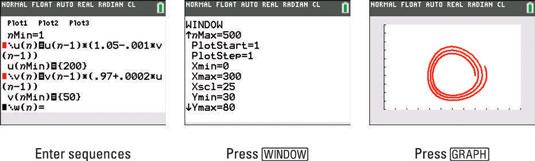##### TI-84 Plus CE Graphing Calculator For Dummies, 3rd EditionIf you want to see how one sequence affects another sequence, you can graph a phase plot on your TI-84 Plus calculator to represent the data.

Enter two sequences that represent the population of rabbits and foxes. Of course, the population of predators is related to the population of its prey.

In the sequence for rabbits: u(n) = u(n–1)*(1.05–0.001*v(n–1), initial condition = 200, 0 < n < 500.

In the sequence for foxes, v(n)=v(n–1)*(0.97+0.0002*u(n–1), initial condition = 50, 0 < n < 500.

Here are the steps to enter and graph a phase plot in your calculator:

1. Press [2nd][ZOOM] to access the Format menu.

2. Use the arrow keys to navigate your cursor to "uv" in the top row and press [ENTER].

3. Press [Y=] to access the Y= editor.

4. Enter the recursive formula for u(n) and v(n).

5. Enter u(nMin) and v(nMin).

Enter the initial term in each sequence as shown in the first screen.6. Press [WINDOW] and adjust the variables.

Make sure your nMax = 500, as shown in the second screen.

7. Press [GRAPH].

See the third screen.